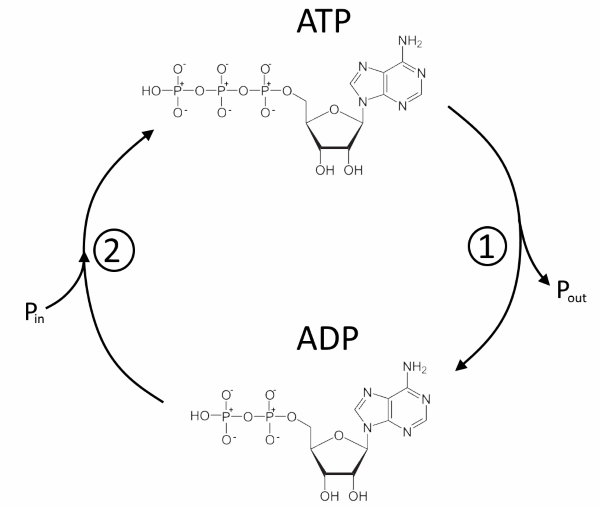﻿Search:

# $ATP$: The battery of lifeThe $ATP$ can be compared to a charged accumulator. His discharge releases energy. The $ADP$ is comparable to an empty accumulator. Its charge consumes energy.

# The structure of $ATP$1 : adenine 2 : ribose 3 : phosphate group Hence the name: AdenosineTriPhosphate

# The reactions of $ATP$## 1: The discharge of the accumulator: Dephosphorylation of $ATP$

This is essentially the case - by hydrolysis : $ATP\;+\;H_2O\;\rightarrow \;H_2PO_4^-\;+\;H^+$ This exergonic hydrolysis ($\Delta G\lt 0$) is not spontaneous because the activation energy is too high. It is often coupled with an enzyme action that lowers this activation energy. - or transfer of the phosphate group : $ATP\;+\;R-OH\;\rightarrow\;R-OPO_3\;+\;$H_2O This transfer, exergonic ($\Delta G \lt 0$) also, is still under the action of enzymes called kinases